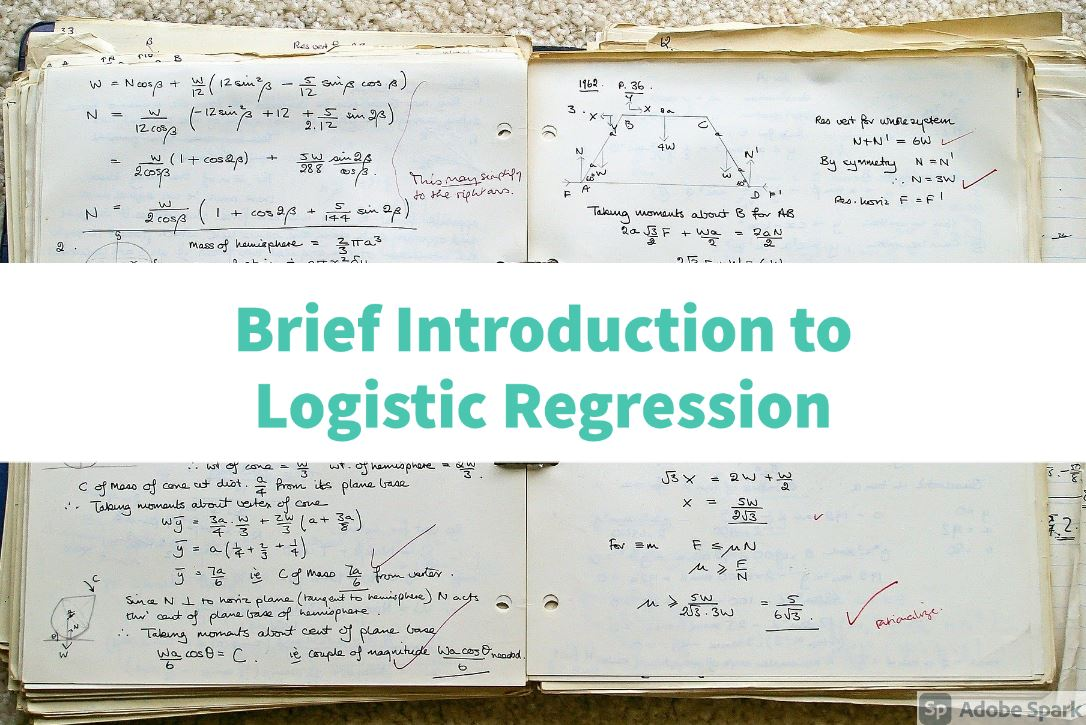# Loops in python

A loop is used for iterating in data structure for example list,tuple,set or a dictionary, also loops can be utilized to iterate in strings.

There are two types of loops in python

1) For loop

2) While loop

### For loop to iterate in list

In this tutorial I am giving simple demonstration of loop to iterate in data structures, and basic overview of how function works. In upcomming tutorial I am gonna teach this stuff in more details.

below is simple demonstration of for loop to iterate in list.

```lst = ["apples","mangoes","oranges","coconut","almonds"]
for value in lst:
print(value)
```

Above piece of script will print all elements in list one by one.

### While loop to iterate in list

Now lets do same thing using while loop

```lst = ["apples","mangoes","oranges","coconut","almonds"]

i = 0
while i<len(lst):
print(lst[i])
i+=1
```

In the same way, we can iterate in tuple using for & while loop.

### Iterating in dictionary

Now lets use for loop to iterate in dictionary

```dictionary = {
0 : "apples",
1 : "mangoes",
2 : "oranges",
3 : "coconut",
4 : "almonds"

}

for keys in dictionary.keys():
print(keys)

for vals in dictionary.values():
print(vals)

for every_thing in dictionary.items():
print(every_thing)
```

As you can see we have used three methods to iterate in dictionary

• First loop will print keys that are 0,1,2,3,4
• Second loop will print values of dictionary that are apples,mangoes,oranges,coconut,almonds
• Third loop will print tuple having keys and values of dictionary for e.g [(0,apples),(1,mangoes),..........]

# Try except block

Lets see basic overview of try except blocks.

Whenever programmers write some code,there are chances that script is going to crash at certain point & when script crashes obviously its gonna stop and normally there are hundreds and thousands of processes going on every time, like on servers or in websites etc.

So try except block helps to catch error and append that error into some log file (if created one to store error messeges) but program will not stop.

 ```1 2 3 4 5 6``` ```try: x = 4 y = 0 print(x/y) except Exception as e: print(str(e)) ```

Now line 4 will cause an error because 4 cant be divided by 0. But as we have used try except block so instead of abortion of script, line number 6 will be executed and it will print error but program will continue to run (if has more lines).

# Functions in python

Functions are containers in which you can write code and call it whenever it is needed. These are useful in reusability of code as well as organizing of the code. each function represent different functionality of program, In this tutorial i have just given basic introduction

but in upcomming tutorial i will teach functions in depth.

to create a function use keyword def and then provide name of function for example

```def main():
pass
```

now to call this function, write

main()

and it will execute all lines in main function.

Now lets create simple python program that will prompt user to input number to check if its prime or not, Program will utlize for loop,while loop, if else, try except and function.

## How to create prime number program in python

```def main():
try:
integer = int(input("Enter number : "))
c = 0
for i in range(2,integer):
if integer%i==0:
c+=1
break
else:
pass
if c==0:
print('Number is prime')
else:
print('Number is not prime')
except Exception as e:
print(str(e))
while True:
main()
```

### Complete Series